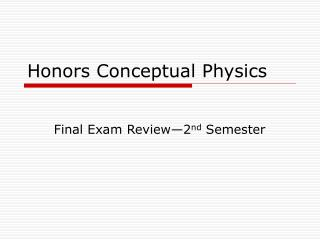Download PresentationHonors Conceptual PhysicsHonors Conceptual Physics - PowerPoint PPT Presentation

Download PresentationHonors Conceptual Physics
An Image/Link below is provided (as is) to download presentation

Download Policy: Content on the Website is provided to you AS IS for your information and personal use and may not be sold / licensed / shared on other websites without getting consent from its author. While downloading, if for some reason you are not able to download a presentation, the publisher may have deleted the file from their server.

- - - - - - - - - - - - - - - - - - - - - - - - - - - E N D - - - - - - - - - - - - - - - - - - - - - - - - - - -
Presentation Transcript

1. Honors Conceptual Physics Final Exam Review—2nd Semester

2. What is the test going to be like? • 92 multiple choice questions. • You will provide your answers on a Scantron bubble sheet. • You will be able to use the test packet to do any scratch work necessary. • You will have the entire period to complete the test (85 minutes). • You must bring a calculator and a pencil! • A formula sheet will be provided.

3. What should I do to prepare? • Complete the study guide. This will help you to identify your strengths and weaknesses. • Get organized: Organize your binder—looking over old materials will help! Get pencils ready, locate a calculator. • Be in class this week!Ask questions while you are in class! • Come in for the review session after school TOMORROW!! (2:35-3:15) • Attend Raider Aid (there is a physics teacher available every day this week!) • Study at home by yourself or with a friend.

4. What do I need to know? • The definitions and units for all vocabulary discussed this semester. • Conceptual ideas related to all topics covered this semester. (Not all questions involve calculations—they test your understanding of the idea) • When and how to use formulas. You will NOT need to memorize them!

5. What do I need to know? • Acceleration: • Identify patterns in graphs and use them to describe the motion of an object. • Position-time, velocity-time and acceleration-time. • Calculate: displacement, distance, time, acceleration and velocity with kinematic equations and graphs.

6. What do I need to know? • Projectile Motion: • Know the rate of acceleration for projectiles. • Know the velocity/acceleration of a projectile at the peak of its path. • Calculate: distance, time, velocity, maximum height, range using formulas. • Use trig to find Vx and Vy.

7. What do I need to know? • Forces: • Identify types of forces present. • Identify correctly drawn FBD’s. • Use trig to calculate components of forces in the x and y direction. • Know the difference between mass and weight. • Calculate weight, force friction and normal force using formulas. • Calculate Fnet using FBD’s. • Know the difference between Coulomb’s Law and the Universal Law of Gravitation.

8. What do I need to know? • Newton’s Law’s • Know the major ideas expressed in all 3 of Newton’s Law’s of motion • Use Newton’s Laws to determine the outcome of a situation including the action of an object or the amount of force experienced by an object. • Calculate Fnet or a using Newton’s 2nd Law.

9. What do I need to know? • Energy: • Identify the 4 major types of energy. • Identify correctly drawn energy bar charts. • Calculate Eg, Ek or Ee using equations. • Calculate Ee using an equation or a graph. • Find the k-value for a spring with a graph (slope) or the F=kx formula. • Find F, x or k using the F=kx formula. • Using energy conservation, find velocity, time, or height for a given object.